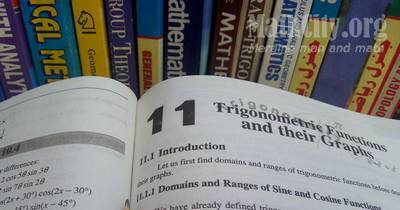# Chapter 11: Trigonometric Functions and their GraphsNotes (Solutions) of Chapter 11: Trigonometric Functions and their Graphs, Text Book of Algebra and Trigonometry Class XI (Mathematics FSc Part 1 or HSSC-I), Punjab Text Book Board, Lahore.

• Introduction
• Domains and Ranges of Sine and Cosine Functions
• Domains and Ranges of Tangent and Cotangent Functions
• Domain and Range of Secant Function
• Domain and Range of Cosecant Function
• Period of Trigonometric Functions
• Exercise 11.1
• Values of Trigonometric Functions
• Graphs of Trigonometric Functions
• Graph of $y = \sin x$ from $-2\pi \hbox{ to } 2\pi$
• Graph of $y = \cos x$ from $-2\pi \hbox{ to } 2\pi$
• Graph of $y = \tan x$ from $-\pi \hbox{ to } \pi$
• Graph of $y = \cot x$ from $-2\pi \hbox{ to } \pi$
• Graph of $y = \sec x$ from $-2\pi \hbox{ to } 2\pi$
• Graph of $y = \csc x$ from $-2\pi \hbox{ to } 2\pi$
• Exercise 11.2

The following short questions was send by Mr. Akhtar Abbas.

• fsc/fsc_part_1_solutions/ch11